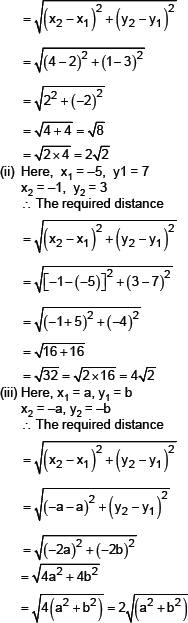# S.K.NEET

## Volume and Surface Area

(i)   441
(ii)   576
(iii)  11025

#### Formulae used :

Distance Formula to find distance between two points (x1,y1) and (x2,y2) is :             D = √[(x2 – x1)2 + (y2 – y1)2 ]

#### Solution :

Sol. (i) Here x1 = 2, y1 = 3, x2 = 4 and y2 = 1
∴ The required distance(i)   441
(ii)   576
(iii)  11025

#### Formulae used :

Distance Formula to find distance between two points (x1,y1) and (x2,y2) is :             D = √[(x2 – x1)2 + (y2 – y1)2 ]

#### Solution :

Sol. (i) Here x1 = 2, y1 = 3, x2 = 4 and y2 = 1
∴ The required distance(i)   1176
(ii)   5625
(iii)  9075

(i)   441
(ii)   576
(iii)  11025

(i)   441
(ii)   576
(iii)  11025

(i)   441
(ii)   576
(iii)  11025

(i)   441
(ii)   576
(iii)  11025

(i)   441
(ii)   576
(iii)  11025

(i)   441
(ii)   576
(iii)  11025

(i)   441
(ii)   576
(iii)  11025

(i)   441
(ii)   576
(iii)  11025

(i)   441
(ii)   576
(iii)  11025

(i)   441
(ii)   576
(iii)  11025

(i)   441
(ii)   576
(iii)  11025

(i)   441
(ii)   576
(iii)  11025